# RD Sharma Solutions Chapter 11 Constructions Exercise 11.2 Class 10 Maths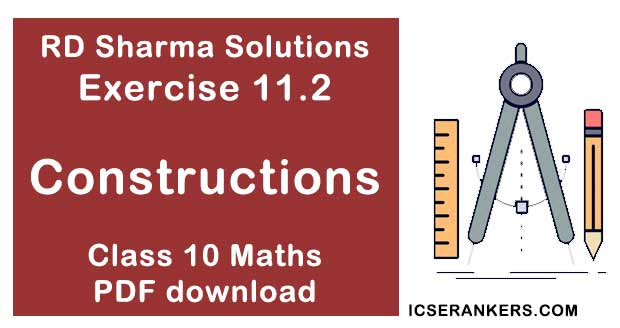Chapter Name RD Sharma Chapter 11 Constructions Book Name RD Sharma Mathematics for Class 10 Other Exercises Exercise 11.1Exercise 11.3 Related Study NCERT Solutions for Class 10 Maths

### Exercise 11.2 Solutions

1. Draw an angle and label it as ∠BAC. Construct another angle, equal  to ∠BAC.

Solution2. Draw an obtuse angle, Bisect it. Measure each of the angles so obtained.

Solution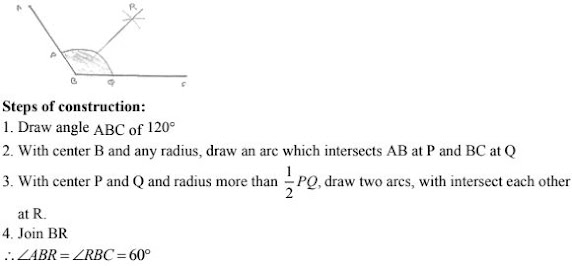3. Using your protractor, draw an angle of measure 108° . With this angle as given, draw an angle of 54°  .

Solution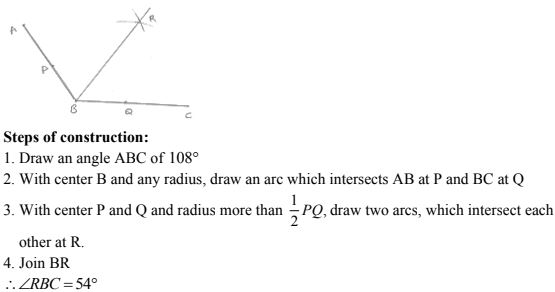4. Using protractor, draw a right angle. Bisect it to get an angle of measure 45° .

Solution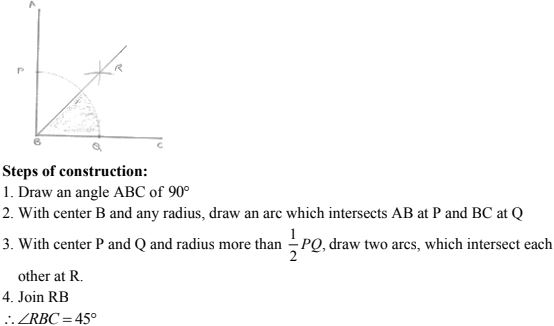5. Draw a linear pair of angles. Bisect each of the two angles. Verify that the two bisecting rays are perpendicular to each other.

Solution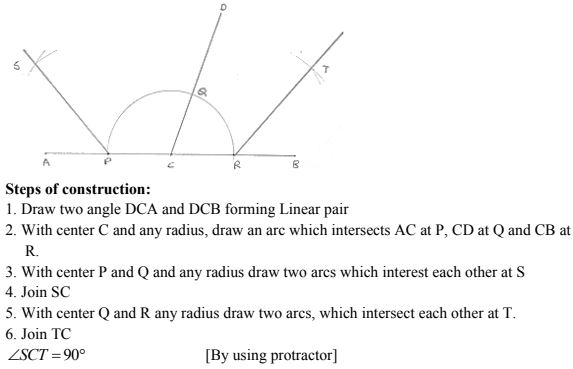6. Draw a pair of vertically opposite angles. Bisect each of the two angles. Verify that the bisecting rays are in the same line.

Solution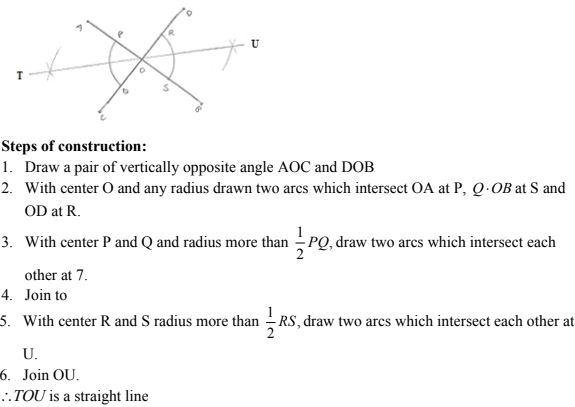7. Using ruler and compasses only, draw a right angle.

Solution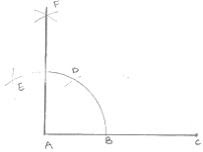Steps of construction :
1. Draw a line segment AB
2. With center A and any radius draw arc which intersect AB at C.
3. With center C and same radius draw an arc which intersects AB at C.
4. With center D and same radius draw arc which intersect arc in (2) at E.
5. With centers E and C and any radius, draw two arcs which intersect each other at F.
6. Join FA
∠FAB = 90°

8. Using ruler and compasses only, draw an angle of measure 135° .

Solution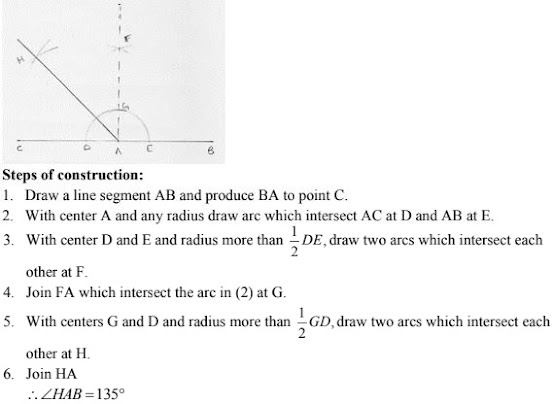9. Using a protractor, draw an angle of measure 72° . With this angle as given, draw angles of measure 36° and 54° .

Solution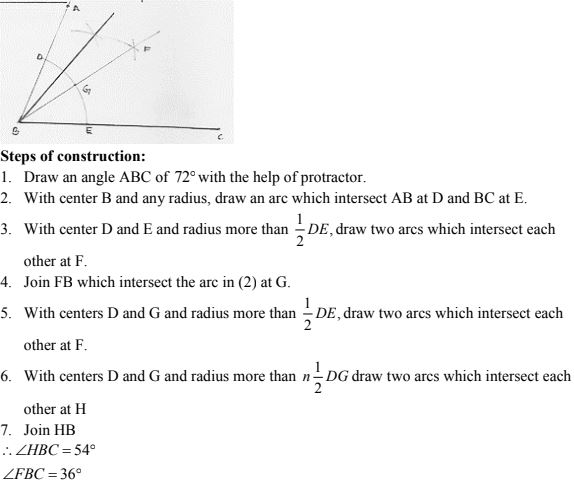10. Construct the following angles at the initial point of a given ray and justify the construction :
(i) 45°  (ii) 90°

Solution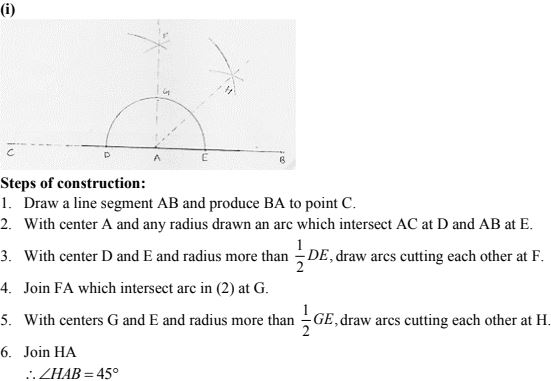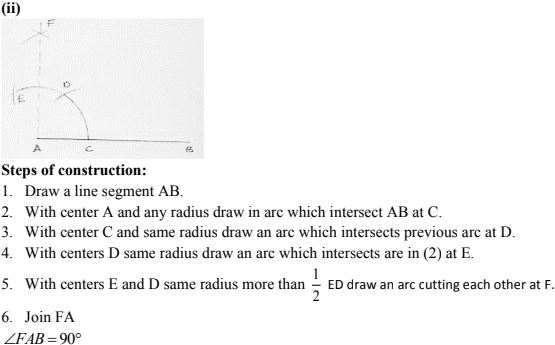11. Construct the angles of the following measurements:
(i) 30°
(ii) 75°
(iii) 105°
(iv) 135°
(v) 15°
(vi) 22(1/2)°

Solution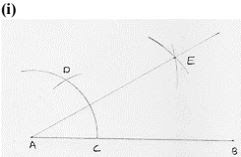Steps of construction:
1. Draw a line segment AB.
2. With center A and any radius, draw an arc which intersect AB at C.
3. With center C and same radius, draw an arc which intersects previous arc at D.
4. With centers D and C and radius more than 1/2 DC, draw arcs intersecting each other at E
5. Join EA
∴ ∠EAB = 30°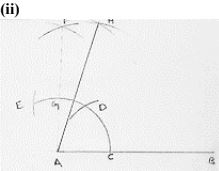Steps of construction:
1. Draw a line segment AB.
2. With center A any radius, draw an arc which intersect AB at C.
3. With center C and same radius, draw an arc which intersects previous arc at D.
4. With center D and same radius, draw an arc which interest are in (2) at E
5. With centers E and D and radius more than 1/2 ED, draw arcs intersecting each other at F.
6. Join FA which intersects arc in (2) at G.
7. With centers G and D, and radius more than 1/2 GD, draw arcs intersecting each other at H.
8. Join HA
∴ ∠HAB = 75°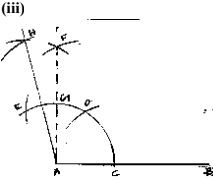Steps of construction:
1. Draw a line segment AB.
2. With center A and any radius, draw an arc which intersect AB at C.
3. With center C and same radius, draw an arc which intersects previous arc at D.
4. With center D and same radius, draw an arc which interest are in (2) at E
5. With centers E and D and radius more than 1/2 ED draw arcs intersecting each other at F.
6. Join FA which intersects arc in (2) at E
7. With centers E and G, and radius more than half of EG, draw arcs intersecting each
other at H.
8. Join HA
∠HAB = 105°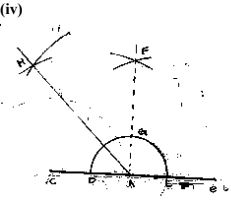Steps of construction:
1. Draw a line segment AB and produce BA to pint C
2. With center A and any radius, draw an arc which intersect AC to D and AB at E.
3. With center D and E and radius more than half of DE, draw two arcs which intersects
each other at F.
4. Join FA which intersect the arc in (2) at G
5. With center G and D radius more than 1/2 GD draw two arcs which intersect each other at H
6. Join HA
∠HAB = 135°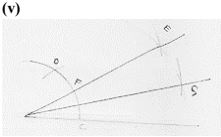Steps of construction:
Step 1: Draw a line segment AB
Step 2: with center A and any radius, draw an arc which intersects previous arc at C
Step 3: with center C and same radius, draw an arc which intersect previous arc at D
Step 4: with center D and C radius more than half of DC draw arcs intersecting each other
at E
Step 5: Join EA which intersects arc in (2) at F.
Step 6: With centers F and C and radius more than 1/2 FC draw arcs intersecting each other
Step 7: Join GA
∠GAB = 15°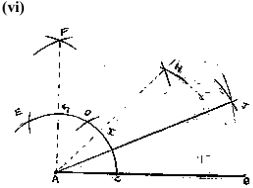Steps of construction:
Step 1: Draw a line segment AB
Step 2: with center A and any radius, draw an arc which intersects AB at C
Step 3: with center C and same radius, draw an arc which intersect previous arc at D
Step 4: with center D and same radius, draw an arc which intersects arc in (2) at E.
Step 5: with center E and D and radius more than half of ED, draw arcs intersecting each other at F.
Step 6: Join FA which intersects arc in (2) at G
Step 7: with center G and C and radius more than half of GC, draw arcs intersecting each other at H
Step 8: Join HA which intersects arc in (2) at 1.
Step 9: with centers I and C and radius more than half of IC, draw arcs intersecting each other
Step 10: Join JA
∴ ∠JAB = 22(1/2)°